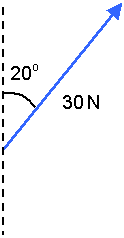##### Vectors and scalarsThe quantities measured in Physics may be divided into two groups:

(a) scalars - these are quantities that have magnitude (size) only.
Examples of scalars are length, speed, mass, density, energy, power, temperature, charge, potential difference

(b)vectors - these are quantities that have direction as well as magnitude.
Examples of vectors are displacement, force, torque, velocity, acceleration, momentum, electric current, magnetic flux density, electric field

Scalars may be added together by simple arithmetic but when two or more vectors are added together their direction must be taken into account as well.
A vector may be represented by a line, the length of the line being the magnitude of the vector and the direction of the line the direction of the vector.